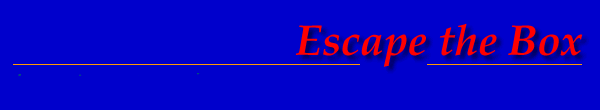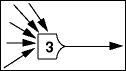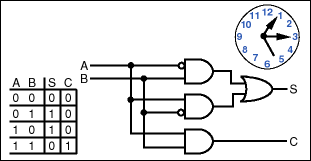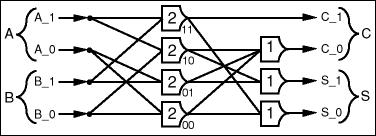Theseus Research : Technical Papers : NULL Convention Logic™ Page 8 of 26Figure 5. Threshold gate.

The most direct way to see how discrete threshold gates combine to make an effective logic circuit is via an example. The half adder will serve as the example and relate the single data value form of NULL Convention Logic to traditional Boolean logic. Figure 6 shows a conventional Boolean logic half-adder circuit with its clock, and Figure 7 shows a NULL Convention Logic half-adder circuit.Figure 6. Boolean logic half-adder circuit with clock.Figure 7. NULL Convention half-adder circuit.

The Boolean half-adder circuit expresses four possible input data values with two data values (0, 1) on two wires (A, B). The NULL Convention half-adder circuit expresses the same four possible input data values with one data value (DATA) on four wires (A_0, A_1, B_0, B_1). Each binary data value A, B, C and S is expressed by a mutually exclusive assertion group of two wires (A_0, A_1 and B_0, B_1 and C_0, C_1 and S_0, S_1). Only one wire in each group can assert DATA at a time, so a complete input data set for the circuit is two DATA values, one from each group (A and B). The completeness of input criteria for each threshold 2 gate is two DATA values. It can be seen that for any threshold 2 gate to assert a data result value there must be one DATA value asserted in each input group and that only one threshold 2 gate at a time will assert a result data value as long as the mutually exclusive assertion convention is enforced for the input groups. The gates, as well as the circuit as a whole, enforce the completeness of input criteria for data. When the result values transition from NULL to a complete result data set, which in this case is one DATA value for each result group (C and S), then the asserted result values are a correct resolution of a complete input data set. The completeness of input criteria for each gate scales up for the circuit as a whole and as before, the completeness of resolution can be determined by simply monitoring the result values. Figure 8 shows the circuit behavior for all four possible input combinations. Bold lines are asserting DATA and thin lines are asserting NULL.